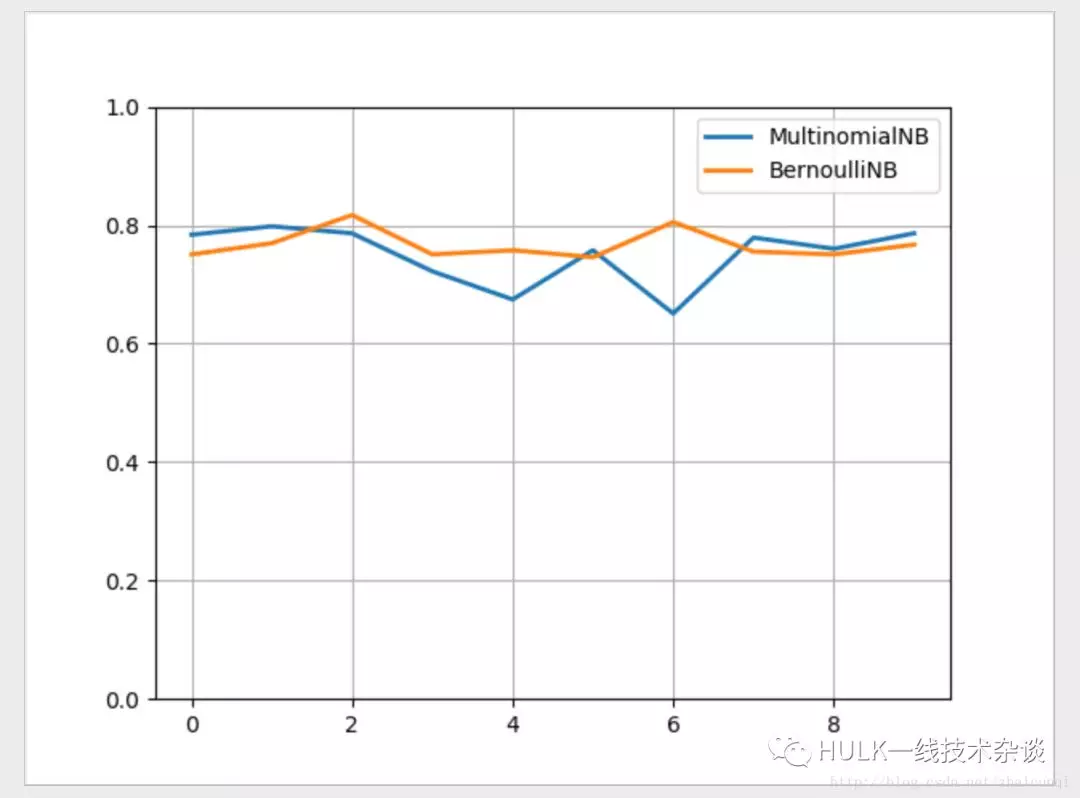# 一起学习朴素贝叶斯## 算法原理

• 条件概率公式
• 全概率公式
• 特征条件独立假设

### 1 条件概率公式

P(A|B) = P(AB)/P(B)P(B|A) = P(AB)/P(A)所以：P(A|B) = P(B|A) * P(A) / P(B)

### 2 全概率公式

P(B) = P(A1B) + P(A1B) + ··· + P(AnB)     = ∑P(AiB)     = ∑P(B|Ai) * P(Ai) ·······················（i=1，2，····，n）

### 3 贝叶斯公式

P(Ak|B) = [ P(Ak) * P(B|Ak) ] / ∑P(B|Ai) * P(Ai） ·········（i=1，2，····，n）

P(Ak|B) = P(Ak) * P(B|Ak)

P(Ak) 先验概率，P(Ak|B) 后验概率，P(B|Ak) 似然函数

## 拉普拉斯平滑

P(y) = (|Dy| + 1) / (|D| + N)

P(xi|Dy) = (|Dy,xi| + 1) / (|Dy| + Ni)

## 文本分类

### 手动实现邮件分类

P(Spam|mail) = P(Spam) * ∏P(wi|Spam)

P(Normal|mail) = P(Normal) * ∏P(wi|Normal)

### 使用 sklearn 实现文本分类

# sklearn 实现文本分类import osimport random from numpy import *from numpy.ma import arangefrom sklearn.pipeline import Pipeline# TfidfVectorizer 文本特征提取（根据词出现的频率及在语句中的重要性）# HashingVectorizer 文本的特征哈希# CountVectorizer 将文本转换为每个词出现的个数的向量 from sklearn.feature_extraction.text import TfidfVectorizerfrom sklearn.naive_bayes import BernoulliNBfrom sklearn.naive_bayes import MultinomialNBimport matplotlib.pyplot as plt # 获取样本集def get_dataset():    data = []    for root, dirs, files in os.walk(r'./mix20_rand700_tokens_cleaned/tokens/neg'):        for file in files:            realpath = os.path.join(root, file)            with open(realpath, errors='ignore') as f:                data.append((f.read(), 'bad'))    for root, dirs, files in os.walk(r'./mix20_rand700_tokens_cleaned/tokens/pos'):        for file in files:            realpath = os.path.join(root, file)            with open(realpath, errors='ignore') as f:                data.append((f.read(), 'good'))    random.shuffle(data)    return data    # 处理训练集与测试集 def train_and_test_data(data_):    # 训练集和测试集的比例为 7:3    filesize = int(0.7 * len(data_))    # 训练集    train_data_ = [each for each in data_[:filesize]]    train_target_ = [each for each in data_[:filesize]]    # 测试集    test_data_ = [each for each in data_[filesize:]]    test_target_ = [each for each in data_[filesize:]]    return train_data_, train_target_, test_data_, test_target_    """多项式模型：在多项式模型中， 设某文档 d=(t1,t2,…,tk)，tk 是该文档中出现过的单词，允许重复，则先验概率 P(c)= 类 c 下单词总数 / 整个训练样本的单词总数类条件概率 P(tk|c)=(类 c 下单词 tk 在各个文档中出现过的次数之和 +1)/(类 c 下单词总数 +|V|)V 是训练样本的单词表（即抽取单词，单词出现多次，只算一个），|V|则表示训练样本包含多少种单词。 P(tk|c) 可以看作是单词 tk 在证明 d 属于类 c 上提供了多大的证据，而 P(c) 则可以认为是类别 c 在整体上占多大比例 (有多大可能性)。"""def mnb(train_da, train_tar, test_da, test_tar):    nbc = Pipeline([        ('vect', TfidfVectorizer()),        ('clf', MultinomialNB(alpha=1.0)),    ])    nbc.fit(train_da, train_tar)  # 训练我们的多项式模型贝叶斯分类器    predict = nbc.predict(test_da)  # 在测试集上预测结果    count = 0  # 统计预测正确的结果个数    for left, right in zip(predict, test_tar):        if left == right:            count += 1    # print(" 多项式模型：", count / len(test_target))    return count / len(test_tar) """伯努利模型：P(c)= 类 c 下文件总数 / 整个训练样本的文件总数P(tk|c)=(类 c 下包含单词 tk 的文件数 +1)/(类 c 下单词总数 +2)"""def bnb(train_da, train_tar, test_da, test_tar):    nbc_1 = Pipeline([        ('vect', TfidfVectorizer()),        ('clf', BernoulliNB(alpha=1.0)),    ])    nbc_1.fit(train_da, train_tar)  # 训练我们的多项式模型贝叶斯分类器    predict = nbc_1.predict(test_da)  # 在测试集上预测结果     count = 0  # 统计预测正确的结果个数    for left, right in zip(predict, test_tar):        if left == right:            count += 1    # print(" 伯努利模型：", count / len(test_target))    return count / len(test_tar) # 训练十次x = arange(10)y1 = []y2 = []for i in x:    print(i)    data = get_dataset()    train_data, train_target, test_data, test_target = train_and_test_data(data)    y1.append(mnb(train_data, train_target, test_data, test_target))    y2.append(bnb(train_data, train_target, test_data, test_target)) print(x)print(y1)print(y2) plt.plot(x, y1, lw='2', label='MultinomialNB')plt.plot(x, y2, lw='2', label='BernoulliNB')plt.legend(loc="upper right")plt.ylim(0, 1)plt.grid(True)plt.show()

sklearn 结果对比## 总结

Scikit learn 也简称 sklearn, 是机器学习领域当中最知名的 python 模块之一。Sklearn 把所有机器学习的模式整合统一起来了，学会了一个模式就可以通吃其他不同类型的学习模式。

https://mp.weixin.qq.com/s/maY1fp341KpJ3mMF1vefJQ